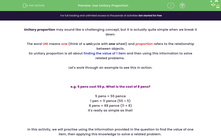# Use Unitary Proportion

In this worksheet, students will practise using the information provided in the question to find the value of one item, then applying this knowledge to solve a related problem.Key stage:  KS 4

Year:  GCSE

GCSE Subjects:   Maths

GCSE Boards:   AQA, Eduqas, Pearson Edexcel, OCR,

Curriculum topic:   Ratio, Proportion and Rates of Change

Curriculum subtopic:   Ratio, Proportion and Rates of Change Direct and Inverse Proportion

Difficulty level:#### Worksheet Overview

Unitary proportion may sound like a challenging concept, but it is actually quite simple when we break it down.

The word UNI means one (think of a unicycle with one wheel) and proportion refers to the relationship between objects.

So unitary proportion is all about finding the value of 1 item and then using this information to solve related problems.

Let's work through an example to see this in action.

e.g. 5 pens cost 55 p. What is the cost of 8 pens?

5 pens = 55 pence

1 pen = 11 pence (55 ÷ 5)

8 pens = 88 pence (11 × 8)

It's really as simple as that!

In this activity, we will practise using the information provided in the question to find the value of one item, then applying this knowledge to solve a related problem.

### What is EdPlace?

We're your National Curriculum aligned online education content provider helping each child succeed in English, maths and science from year 1 to GCSE. With an EdPlace account you’ll be able to track and measure progress, helping each child achieve their best. We build confidence and attainment by personalising each child’s learning at a level that suits them.

Get started